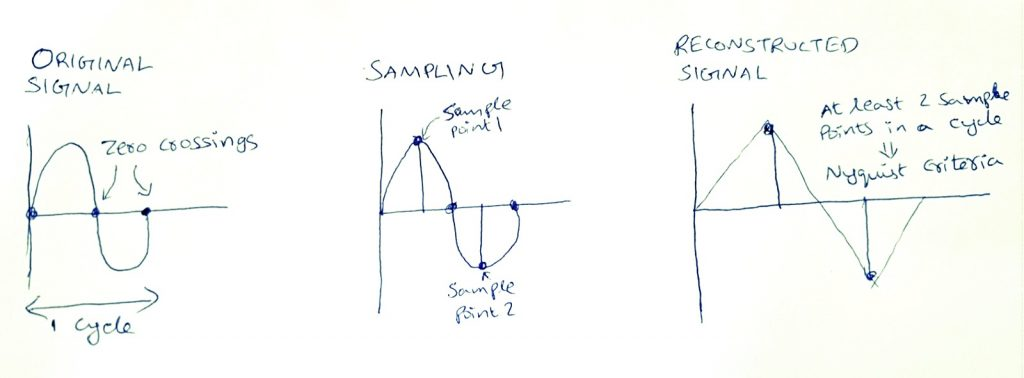# Frequencies•October 25, 2016
•3 mins read

I would like to continue the blog series I started earlier – Introducing Digital Audio – with this story about frequencies and elucidating what they mean. Frequency is a word that one frequently encounters when they read any book or research paper related to digital signal processing on any multimedia. And, when it comes to audio, there are different types of frequencies.

To begin with, let us consider an original analog signal. The amplitude of an analog signal varies in the timeline between positive values and negative values. When the wave crosses the zero amplitude 2 times, it means that it finishes a cycle. The time it took to complete a cycle is time-period of the signal (measured in seconds), and the inverse of time-period is the frequency of the signal (measured in Hertz). If the time-period is constant for all the cycles (ex: perfect sine wave), it means that there is only one single frequency in that signal. But speech and music constitute multiple frequencies, and the range of frequencies a signal has significant energy is called the spectrum of the signal.

Let us jump to digital domain now. When the analog signal is sampled (discrete values in time) and quantized (discrete values in amplitude), we will get a digital signal which is nothing but a 1-D array of sample amplitude values (real integers). This digital signal has a digital frequency which depends on original analog signal frequency and sampling frequency. The digital frequency or the normalized frequency is nothing but the original analog frequency (every signal has primary frequency even though it contains a band of frequencies) divided by the sampling frequency.

The sampling frequency is determined by Nyquist criteria. Nyquist criteria states that to faithfully reproduce original analog frequency from the digitized signal (sampled & quantized), at least 2 sample values inside a cycle (time period of the signal) is needed. That is, sampling frequency should be at least twice that of original signal frequency.

Equation:

fs >= 2*fFrom the equation above, Nyquist frequency is defined as half the sampling frequency; that is fN = 0.5 * fs. Nyquist criteria is very important for sampling, otherwise, it would result in temporal aliasing effect in audio. To understand the aliasing effect, one must know that the frequency which constitutes the high energy in the spectrum is called fundamental frequency and integer multiples of the fundamental frequency are called harmonics. So, usually the signal is low pass filtered with high cut-off frequency as the fundamental frequency of this signal. This filter is called anti-alias filter as it filter outs the energies from higher order harmonics and preserves the original spectrum even after sampling.

Consider an example of a perfect sinusoidal wave of 10KHz frequency. Then integer multiples of that fundamental frequency such as 20 KHz, 30 KHz, 40 KHz, etc. are higher order harmonics or alias frequencies. This is because a signal making 1 complete cycle in 10 KHz also makes 1 complete cycle in 20 KHz if the sampling rate is halved. This is why it is important to find the fundamental frequency to avoid problems of oversampling and undersampling.

From the digital samples, the frequency can be determined. Since the amplitude and frequency of audio signals vary considerably, it is always better to calculate the frequencies for smaller windows like 10 ms frame of audio. The less accurate way to predict frequency for a 10 ms frame is by counting the number of “zero-crossings” in the sample values. Another way is to measure using Fast Fourier Transform (FFT). FFT returns an array of complex numbers where the real part signifies the amplitude and the imaginary part signifies the phase. The frequency in Hertz can be determined by the peak absolute value of the array and by multiplying it by the sample rate (that is the number of samples per second).

It is important to understand that both the amplitude and the frequency varies in the log scale in digital audio. That’s why amplitude and energies are usually measured in dB scale. Signal power is RMS (Root Mean Square) value of samples in a window measured in log scale. When signal power is plotted against frequencies, it gives power spectrum of the signal. When both signal power and frequencies are plotted in log scale in a power spectrum it is called cepstrum or Mel scale. Mel frequency defines the log frequency ranges to measure signal power or energy. The total energy contained within each Mel-scale frequency bands are called logbank filter energies. Taking inverse transform over logbank filter energies (to decorrelate) give Mel Frequency Cepstral Coefficients (MFCC). This MFCC is a very important feature used in Automatic Speech Recognition (ASR).

I will continue this series of post with next article on processing the digital audio data.